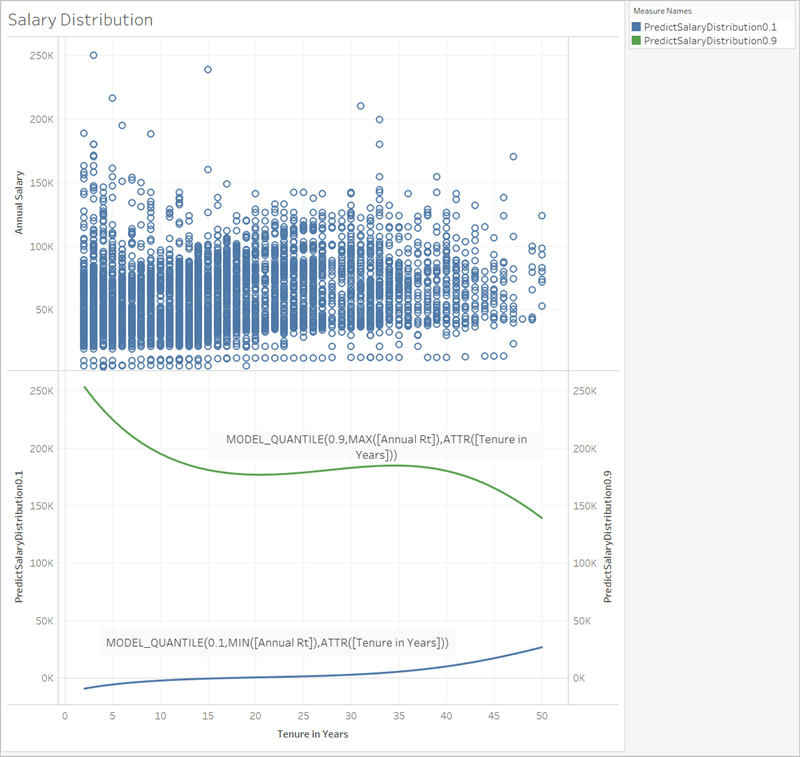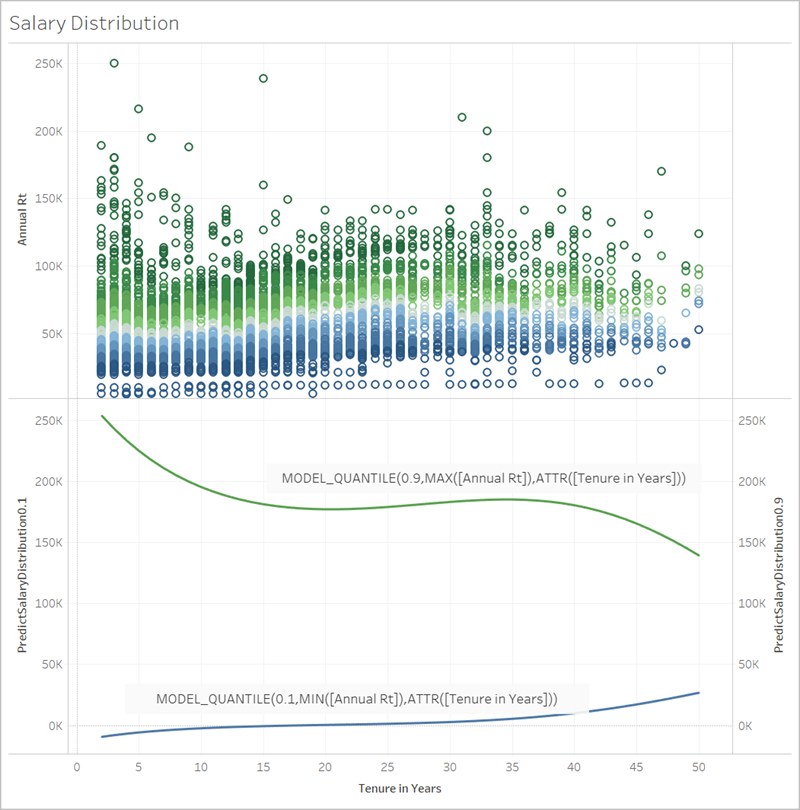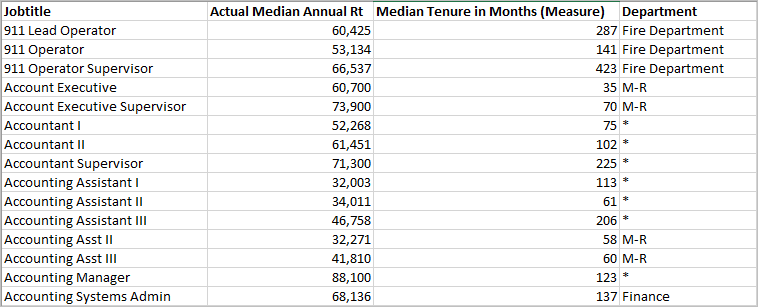You can already add trend lines and forecasts to a visualisation, but now you can go beyond this, using the power of a statistical engine to build a model that understands how your data is distributed around such a trend line, or line of best fit. Previously, users had to integrate Tableau with R and Python in order to perform advanced statistical calculations and visualise them in Tableau. Now, you can use the predictive modelling functions to make predictions from your data by including them in a table calculation. For more information about table calculations, see Transform Values with Table Calculations.

With these predictive modelling functions, you can select targets and predictors by updating the variables and visualising multiple models with different combinations of predictors. The data can be filtered, aggregated and transformed at any level of detail, and the model – and thus the prediction – will automatically recalculate to match your data.

For a detailed example that shows how to create prediction calculations using these functions, see Example – Explore Female Life Expectancy with Predictive Modelling Functions.

## Predictive modelling functions available in Tableau

 Function Syntax Description MODEL_QUANTILE ```MODEL_QUANTILE( model_specification (optional), quantile, target_expression, predictor_expression(s))``` Returns a target numeric value within the probable range defined by the target expression and other predictors, at a specified quantile. This is the Posterior Predictive Quantile.Example:`MODEL_QUANTILE(0.5, SUM([Sales]),COUNT([Orders]))` MODEL_PERCENTILE ```MODEL_PERCENTILE( model_specification (optional), target_expression, predictor_expression(s))``` Returns the probability (between 0 and 1) of the expected value being less than or equal to the observed mark, defined by the target expression and other predictors. This is the Posterior Predictive Distribution Function, also known as the Cumulative Distribution Function (CDF).Example:`MODEL_PERCENTILE( SUM([Sales]),COUNT([Orders]))`

## The power of predictive modelling functions

Let’s look at an example using salary data, starting with MODEL_QUANTILE.

In the example below, MODEL_QUANTILE has been used to display the 10th percentile and the 90th percentile of the expected distribution for the same data set. Based on the existing data and using a linear regression model, the statistical engine has determined that there is a 90% probability that the maximum salary for each tenure will be below the green line, and a 10% probability that the minimum salary for each tenure will be below the blue line.

In other words, with the quantile set at 0.9, the model predicts all salaries will fall at or below the green line 90% of the time. The blue line is set at .1 or 10%, so only 10% of salaries will fall at or below the blue line, with the inverse of that (90%) above the blue line.

Effectively, this gives us a band that we can predict 80% of potentially generated future points or unobserved data will fall inside.Next, let’s see how MODEL_PERCENTILE, the inverse of MODEL_QUANTILE, can help us understand the data further.

You can identify the outliers of the data set by using the MODEL_PERCENTILE function. MODEL_PERCENTILE tells you, as a percentile, where the observed mark falls within a range of probable values for each mark. If the percentile is very close to 0.5, the value observed is very close to the median value predicted. If the percentile is close to 0 or 1, the value observed is at the lower or upper boundaries of the model range and is relatively unexpected.

Below, we have applied MODEL_PERCENTILE as colour to the salary visualisation in the top half of the image, to help us understand which values are most expected.## Syntax of predictive modelling functions in detail

### What is MODEL_QUANTILE?

MODEL_QUANTILE calculates the posterior predictive quantile, or the expected value at a specified quantile.

• Quantile: The first argument is a number between 0 and 1, indicating what quantile should be predicted. For example, 0.5 specifies that the median will be predicted.
• Target expression: The second argument is the measure to predict or ‘target’.
• Predictor expression(s): The third argument is the predictor used to make the prediction. Predictors can be dimensions, measures or both.

The result is a number within the probable range.

You can use MODEL_QUANTILE to generate a confidence interval, missing values such as future dates, or to generate categories that don't exist in your underlying data set.

### What is MODEL_PERCENTILE?

MODEL_PERCENTILE calculates the posterior predictive distribution function, also known as the Cumulative Distribution Function (CDF). This calculates the quantile of a particular value between 0 and 1, the inverse of MODEL_QUANTILE.

• Target expression: The first argument is the measure to target, identifying which values to assess.
• Predictor expression(s): The second argument is the predictor used to make the prediction.
• Additional arguments are optional and are included to control the prediction.

Notice that the calculation syntax is similar, with MODEL_QUANTILE having the extra argument of a defined quantile.

The result is the probability of the expected value being less than or equal to the observed value expressed in the mark.

You can use MODEL_PERCENTILE to surface correlations and relationships within your database. If MODEL_PERCENTILE returns a value close to 0.5, the observed mark is near the median of the range of predicted values, given the other predictors that you've selected. If MODEL_PERCENTILE returns a value close to 0 or to 1, the observed mark is near the lower or upper range of what the model expects, given the other predictors that you've selected.

Note: Dimensions used as predictors can be ordered or unordered. An ordered dimension is any dimension whose values can be sequenced, such as MONTH. An unordered dimension is any dimension whose values don't have an inherent sequence, such as gender or colour. This distinction matters when using Gaussian process regression. For more information on this model and others, see Choosing a Predictive Model.

For advanced users, there are two other optional arguments you can include to control the prediction. For more information, see Regularisation and Augmentation in Predictive Modelling.

## What’s being computed?

The input used to build the model is a matrix where each mark is a row, and the columns are the target expression and predictor expressions evaluated for each mark. Whatever row is specified on the viz is what defines the row for the data set being computed by the statistical engine.

Let’s look at the example below, where rows (and thus marks) are defined by job titles, and columns are the target expression MEDIAN([Annual Rt]). These are followed by the optional additional predictors MEDIAN([Tenure in Months (Measure)] and ATTR([Department Generic (group)].Note: Several job titles have an asterisk listed under Department. This is because those job titles exist in several departments, but Tableau treats all of them as though they are in the same department. In addition, the statistical engine doesn’t consider the number of records, and treats each mark as equally likely. This is because Tableau can’t perform table calculations on unaggregated data, and can’t aggregate the results of table calculations. For more information on aggregation, see Data Aggregation in Tableau.

For more information on the data used to build a model and generate predictions, see Compute Using and Data Partitioning in Predictive Modelling.

## What models are supported?

Predictive modelling functions support linear regression, regularised linear regression and Gaussian process regression. These models support different use cases and prediction types, as well as having different limitations. For more information, see Choosing a Predictive Model.

A predictor can be any field in your data source – measure or dimension – including calculated fields.

Note: If using a dimension as a predictor, you must use a ATTR wrapper (e.g. ATTR([State]) rather than just [State]). This is because dimensions will generally only work if they are in the level of detail in the visualisation either directly, or above it in a hierarchy.

For example, suppose you have a data set that includes the fields [City], [State] and [Region], where multiple[City] records exist within a [State], and multiple [State] records within a [Region].

In a visualisation that uses [State] as a mark, the predictor ATTR([State]) or ATTR([Region]) will both work as predictors. However, the predictor ATTR([City]) will revert to *, as multiple cities exist within a visualised state and so cannot be used as predictors. In other words, including a predictor that's at a lower level of detail than the viz adds no value to the predictions. In most cases, a predictor at a lower level of detail than the viz will evaluate to * and so will all be treated identically.

However, if the same data set is used to generate a viz that uses [City] as a mark, ATTR([City]), ATTR([State]) and ATTR([Region]) can all be used successfully as predictors. For more information about the use of ATTR functions, see When to Use the Attribute (ATTR) Function.

Dimensions and measures do not need to be visualised (in the view or viz) in order to be included as predictors. For more detailed guidance, see Choosing Predictors.

## Recommendations

Prediction calculations are best used as follows:

• To predict values for individual records, where each mark in your viz represents a discrete entity such as a product, sale, person, etc., rather than aggregated data. This is because Tableau regards each mark as equally likely, even if one mark is made up of 100 records and the other marks are made up of one record each. The statistical engine does not weigh marks based on the number of records that make it up.

• To predict values for aggregated target expressions using SUM and COUNT.
Note: MODEL_QUANTILE and MODEL_PERCENTILE aren’t recommended to predict values for aggregated target_expressions using AVG, MEDIAN, MIN and MAX.

## Limitations

• You must use a calculated field to extend a time series into the future. For more information, see Predicting the Future.

• Predictors should be at the same or higher level of detail than the view. That is, if your view aggregates by state, you should use state or region as a predictor, but not city. For more information, see Choosing Predictors.

## When will prediction calculations break?

Regardless of the model you're using, you must have at least three data points within each partition for the model to return a response.

If you have specified Gaussian process regression as your model, it can be used in predictive calculations with one ordered dimensional predictor and any number of unordered dimensional predictors. Measures aren't supported as predictors in Gaussian process regression calculations, but can be used in linear and regularised linear regression calculations. For more information on model selection, see Choosing a Predictive Model.

If your calculation used ATTR[State] as a predictor and your viz also included State as a mark but no other field at a lower level of detail, such as City, then you would return an error. To prevent this, simply ensure there is not a one-to-one relationship between marks and predictor categories.

For more information on these and other prediction problems, see Resolve Errors in Predictive Modelling Functions.

## FAQ

### What about marks in multiple predictor-groups?

If a row is aggregated from data that exists within multiple predictor-groups, the value of the ATTR function is a special value of multiple values. For instance, all cities that exist in multiple states will have the same predicted value (unless there are other predictors that are distinct). When selecting your predictors, it's best to use predictors that are at the same level of detail as the viz or or higher. Again, for more information on ATTR functions, see When to Use the Attribute (ATTR) Function.

### What if ATTR Aggregation returns a * value?

* is treated as a distinct value. If ATTR returns * for all marks, then you, in essence, have a predictor with a constant value, which will be ignored. This is the same as not including that predictor at all.

If ATTR returns * for some but not all marks, then it will be treated as a category where all the * values are considered to be the same. This scenario is identical to the above scenario, in which marks exist in multiple predictor-groups.